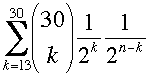SEARCH HOMEMath Central Quandaries & QueriesQuestion from seulki, a student: coin tossed 30 times, what is the probability that the heads show up fewer than 17 times?Hi Seulki,

This is a binomial distribution problem. There is an experiment, tossing a coin, which is repeated 30 times and the probability of tossing a head is 1/2 on each of the 30 trials. Thus for example the probability that the head shows up more than 12 times isI am not sure how you are to evaluate this. You can find each one of the terms and add them, you can use a binomial table or you can use the normal approximation to the binomial.

HarleyMath Central is supported by the University of Regina and The Pacific Institute for the Mathematical Sciences.